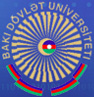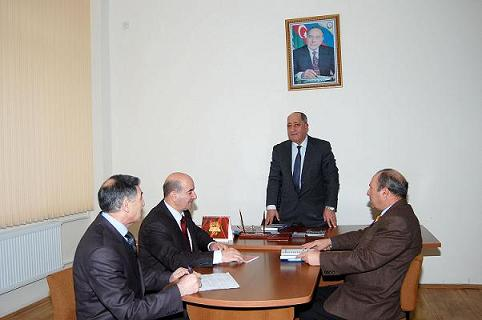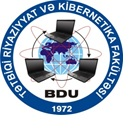## Faculty of Applied Mathematics and Cybernetics

The faculty of Applied Mathematics and Cybernetics (FAMC) where high skilled specialists by specialty "Applied Mathematics", "Information Science" and "Economical Cybernetics" are prepared is formed by the decree and personal patronage of common national leader H. Aliyev in 1972. 17 professors and doctors of science (one of them is a full member, 3 corresponding members of Azerbaijan National Academy of Science), 54 assistant professors and Ph. Doctors pass their knowledge and skills to the young generation - students, masters and postgraduate students. Here four computer classes and a library are operating that creates favorable conditions for profound development of students. Besides that, on the 12th of December, 2008, Azerbaijan-Korea Information Center opened at the faculty which plays an important role in the development of intellectual level of the faculty students.

At the FAMP a special attention is paid to the preparation of scientific-pedagogical staff. In 2008/2009 academic year 95 undergraduate masters 12 of them are citizens of the Iran Islamic Republic (IIR) are getting education by 15 specialties at the faculty. Besides that, 29 postgraduate students (18 of them are from the IIR) and 2 dissertants are engaged with scientific-research work.

Weekly seminars of the FAMC departments are held regularly. Not only fellow workers of the faculty but the ones from the other faculties institutions take part in this seminars .The scientific articles are published in prestigious scientific journals of our Republic and other countries.10 departments are acting at the FAMC :
1. The department of Applied Mathematics
2. The department of Equations of Mathematical Physics
3. The department of Information technologies and programming
4. The department of Mathematical Cybernetics
5. The department of Optimization and Optimal Control
6. The department of Operations Research and Mathematical Modeling
7. The department of Theory of Probability and Mathematical statistics
8. The department of Economic Cybernetics (Mathematical economic and modelling of economy-ecological systems)
9. The department of Mathematical methods of Applied analysis
10. The department of Information Science

Scientific-research work is held at the faculty by the following 17 subjects:
1. Operator-differential operators and spectral problems connected with them.
2. Direct and inverse problems of inverse spectral analysis of differential operations
3. Approximate methods of solving problems of mathematical physics and problems of their convergence.
4. Spectral theory of ordinary differential equations.
5. Investigation of boundary problems for linear and nonlinear differential equations in partial derivatives.
6. Control methods for parallel transactions for one-version and multi-version models of distributed data bases.
7. Analysis and synthesis of consequent machines of discrete and continuous dynamic systems.
8. Discrete optimization, optimal control and theory of games.
9. Identification of nonlinear systems with distributed parameters and models of control.
10. Some methods and models of applied financial analysis.
11. Nonlinear boundary problems for random processes and their application to theory of statistical activities checking.
12. Asymptotic properties of random processes expressing intricate systems.
13. Investigation of fuzzy models of market and creation of software.
14. Mathematical problems of solid mechanics.
15. Some problems of qualitative theory of optimal control
16. Analysis of fuzzy theory application in the problems of taking decisions with economico-ecological decline with fuzzy factors
17. Peculiarities of labour market in the AR and socio-economical aspects of development

The FAMC has connections with some the centers of science and education situated on the territory of former Soviet Union. There may be marked such as Moscow, St-Petersburg, Novosibirsk, Rostov, Kiev, Belarus, Tbilisi, Riga State Universities, Institute of Mathematics named after Steklov of Russian Academy of Sciences, Institute of Mathematics of Siberian department of RAS, Moscow Institute of Energy, Voronezh Pedagogical Institute, Institute of Cybernetics of the Ukrainian Academy of Science, Technical University of Vinitsa (Ukraine), Institute of mathematics in Belarus, and others. There exist relationships with Universities of USA, Germany, Italy, Great Britain, France, Czechia, Israel, Turkey, Iran and others states.
Bonn, Leipzig, Berlin Universities, Zanjan University of the IIR must be particularly pointed out.

Some collaborators of the faculty are working in various Universities of the Turkish Republic by contract.

Undergraduate bachelors are prepared at the FAMC by the following specialties:
1. Theory of Probability and Mathematical statistics.
2. Investigation of operations and system analysis.
3. Mathematical Cybernetics.
4. System programming.
5. Mathematical modeling.
6. Mathematics and Information software of economical activity.
7. Software of calculating networks.
8. Mathematical Physics.
9. Optimization and optimal control.
10. Calculating diagnostics.
11. Mathematical-economical methods of optimal control.
12. Information Science.
13. Discrete systems.
14. Analysis of complex systems by probability and Statistical methods.
15. Economical Cybernetics

Principal scientific-research branches:
1. Direct and inverse problems of spectral analysis of differential operators
2. Operator-differential equations and spectral problems connected with them
3. Approximate problems of solving of mathematical physics and problems of their convergence
4. Spectral theory of ordinary differential equations
5. Investigation of boundary problems for linear and nonlinear differential equations in partial derivatives
6. Optimal identification of nonlinear systems.
7. Discrete dynamic systems and theory of consequent machines
8. Discrete optimization and optimal control
9. Models of applied functional analysis
10. Systems of mass service, theory of reserves control, theory of random walk, problems of regress, statistical modeling and statistical analysis
11. Theoretical and practical problems of applied processes mutual connection in computer nets.

Logo faculty